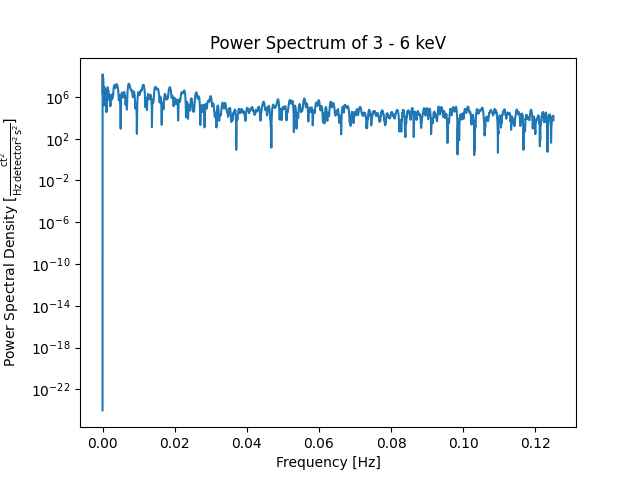# Making a power spectrum from a TimeSeries¶

How to estimate the power spectrum of a TimeSeries.

```import matplotlib.pyplot as plt
from scipy import signal

import astropy.units as u

import sunpy.timeseries
from sunpy.data.sample import RHESSI_TIMESERIES
```

Let’s first load a RHESSI TimeSeries from sunpy’s sample data. This data contains 9 columns, which are evenly sampled with a time step of 4 seconds.

```ts = sunpy.timeseries.TimeSeries(RHESSI_TIMESERIES)
```

We now use SciPy’s `periodogram` to estimate the power spectra of the first column of the Timeseries. The first column contains X-Ray emissions in the range of 3-6 keV. An alternative version is Astropy’s `LombScargle` periodogram.

```x_ray = ts.columns
# The suitable value for fs would be 0.25 Hz as the time step is 4 s.
freq, spectra = signal.periodogram(ts.quantity(x_ray), fs=0.25)
```

Let’s plot the results.

```plt.figure()
plt.semilogy(freq, spectra)
plt.title(f'Power Spectrum of {x_ray}')
plt.ylabel('Power Spectral Density [{:LaTeX}]'.format(ts.units[x_ray] ** 2 / u.Hz))
plt.xlabel('Frequency [Hz]')
plt.show()
```Total running time of the script: ( 0 minutes 0.636 seconds)

Gallery generated by Sphinx-Gallery